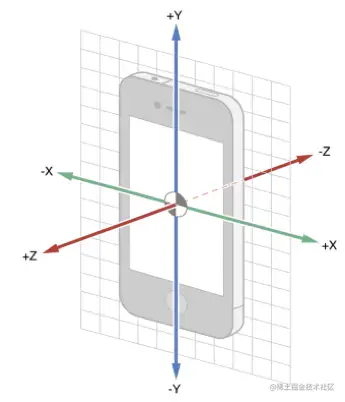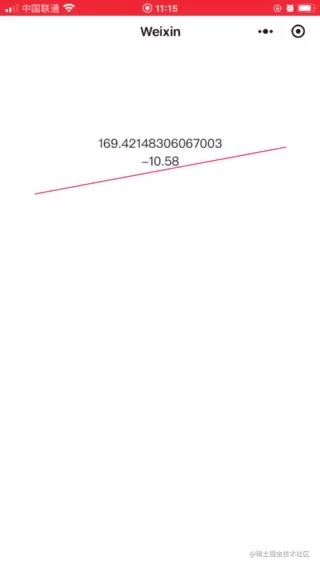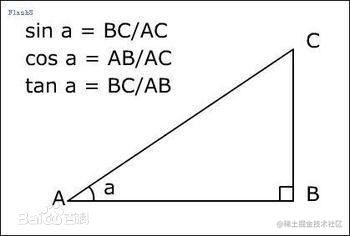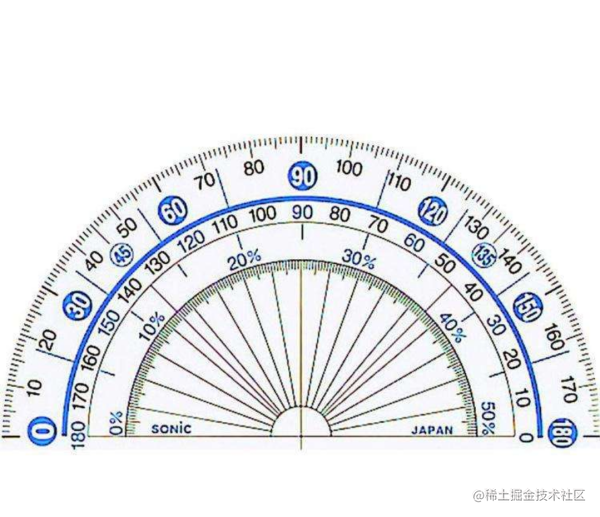# 小程序的加速计的接口

wx.onAccelerometerChange(function callback)

function callback

Object res

x number X 轴
y number Y 轴
z number Z 轴

``````  wx.onAccelerometerChange(function (res) {
const { x,y,z} = res;
})
``````

# 手机的xyz轴# 数学知识

## 如上图，这样我们只要计算x与y的夹角就可以了，接下来怎么计算呢，我们用到两个数学知识

1. Math.atan2()** Math.atan2()接受两个参数x和y,方法如下:**

• angel=Math.atan2(y,x)

• x 指定点的 x 坐标的数字。

• y 指定点的 y 坐标的数字。

• 计算出来的结果angel是一个弧度值,也可以表示相对直角三角形对角的角，其中 x 是临边边长，而 y 是对边边长。

**2. 角度=弧度*180/Math.PI``````  wx.onAccelerometerChange(function (res) {
const { x,y,z} = res;
//根据公式计算出角度
const rotateXY = Math.atan2(x,y) * 180 / Math.PI;
// 这里角度就可以赋值到data上，视图层就可以调这个角度了
this.setData({
rotateXY: rotateXY
})
})
``````

``````<view style="width:600rpx; height:2rpx;background:red;transform:rotate({{rotateXY}}deg)">
``````

# 扫码体验